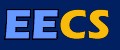# Monte-Carlo Methods for Estimating System Reliability

### http://www.eecs.berkeley.edu/Pubs/TechRpts/1984/CSD-84-168.pdf

An n-component system contains n components, where each component may be either failing or working. Each component i is failing with probability Pi independently of the other components in the system. A system state is a specification of the states of the n components. Let F, the set of failure states, be a specified subset of all system states. In this paper we develop Monte Carlo algorithms to estimate Pr[F], the probability that the system is in a failure state.

We now describe the two different formats for the representation of F considered in this paper. In the first format F is represented by m failure sets F1, F2, . . . , Fm. Each failure set is specified by an n-tuple in the input. The set of failure states is then F=m,t=1Fi. We develop a formula for the probability of a union of events. A Monte Carlo algorithm which estimates the failure probability of an n-component system is developed based on this formula. One trial of the algorithm outputs an unbiased estimator of Pr[F]. Let Y be the average of the estimators produced by many trials of the algorithm. We show that, when the algorithm is run for an amount of time proportional to nm, Y is provably close to Pr[F] with high probability.

The second format for the representation of F can be described as follows. A network is an undirected graph G, where the edges in the graph correspond to the components in the system. Let G be a planar network and let x1, . . . , xK be K specified nodes in G. For the planar K-terminal problem, the network is in a failing state if there is no path of working edges between some pair of specified nodes. We develop a Monte Carlo algorithm to estimate Pr[F] for the planar K-terminal problem. The algorithm works especially well when the edge failure probabilities are small. In this case the algorithm produces an estimator Y which is provably close to Pr[F] with high probability in time polynomial in the size of the graph. This compares very favorably with the execution times of other methods used for solving this problem.

Advisor: Richard M. Karp

BibTeX citation:

```@phdthesis{Luby:CSD-84-168,
Author = {Luby, Michael G.},
Title = {Monte-Carlo Methods for Estimating System Reliability},
School = {EECS Department, University of California, Berkeley},
Year = {1983},
Month = {Jun},
URL = {http://www.eecs.berkeley.edu/Pubs/TechRpts/1983/5978.html},
Number = {UCB/CSD-84-168}
}
```

EndNote citation:

```%0 Thesis
%A Luby, Michael G.
%T Monte-Carlo Methods for Estimating System Reliability
%I EECS Department, University of California, Berkeley
%D 1983
%@ UCB/CSD-84-168
%U http://www.eecs.berkeley.edu/Pubs/TechRpts/1983/5978.html
%F Luby:CSD-84-168
```